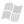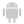WINDEVWEBDEVWINDEV MobileOthersIn french: TableurRécupèreFormule
Retrieves the calculation formula of a cell found in a Spreadsheet control of a window.
Example
Syntax

Retrieving the formula of a cell identified by its name

<Result>: Character string
• Formula in the specified language. This formula does not contain the '=' character.
• Empty string ("") if the cell contains no formula.
Name of the Spreadsheet control to be used.
<Cell>: Character string
Name of cell for which the formula will be retrieved. This parameter has the following syntax: <Column letter><Row number>. For example: "A32".
<Language>: Optional Integer constant
Constant used to specify the language in which the formula will be retrieved.
• If this parameter corresponds to the nationFrench constant, the formula is retrieved in French.
• If this parameter is not specified or if it differs from the nationFrench constant, the formula si retrieved in English (native Excel format).

Retrieving the formula of a cell identified by its coordinates

<Result>: Character string
• Formula in the specified language. This formula does not contain the '=' character.
• Empty string ("") if the cell contains no formula.
Name of the Spreadsheet control to be used.
<Column>: Integer
Number of the column corresponding to the cell for which the formula must be retrieved.
<Row>: Integer
Number of the row corresponding to the cell for which the formula must be retrieved.
<Language>: Optional Integer constant
Constant used to specify the language in which the formula will be retrieved.
• If this parameter corresponds to the nationFrench constant, the formula is retrieved in French.
• If this parameter is not specified or if it differs from the nationFrench constant, the formula si retrieved in English (native Excel format).
Related Examples:Unit examples (WINDEV): The Spreadsheet control [ + ] Using the Spreadsheet control.This example explains how to:- load an xlsx file in a spreadsheet control,- save the spreadsheet in a file,- fill the control with data coming from the database,- insert rows, columns,- access the cells and handle them (modify their value, their style, ...),- enter formulas,- ...
Component: wd290obj.dll
Minimum version required
• Version 20